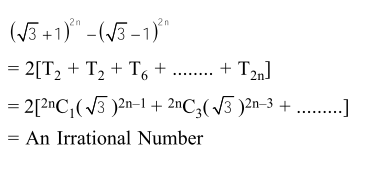# If n is a positive integer, then`
Question:

If $\mathrm{n}$ is a positive integer, then $(\sqrt{3}+1)^{2 \mathrm{n}}-(\sqrt{3}-1)^{2 \mathrm{n}}$ is :

1.  a rational number other than positive integers

2. an irrational number

3. an odd positive integer

4. an even positive integer

Correct Option: , 2

Solution: